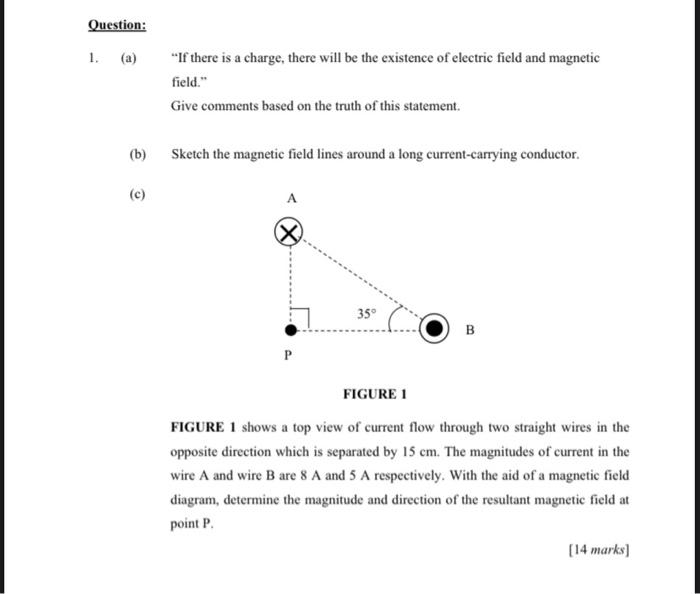# Question 1. (a) "If there is a charge, there will be the existence of electric field and magnetic field." Give comments based on the truth of this statement. (b) Sketch the magnetic field lines around a long current-carrying conductor. (c) FIGURE 1 FIGURE 1 shows a top view of current flow through two straight wires in the opposite direction which is separated by $$15 \mathrm{~cm}$$. The magnitudes of current in the wire $$\mathrm{A}$$ and wire $$\mathrm{B}$$ are $$8 \mathrm{~A}$$ and $$5 \mathrm{~A}$$ respectively. With the aid of a magnetic field diagram, determine the magnitude and direction of the resultant magnetic field at point $$P$$.Transcribed Image Text: 1. (a) "If there is a charge, there will be the existence of electric field and magnetic field." Give comments based on the truth of this statement. (b) Sketch the magnetic field lines around a long current-carrying conductor. (c) FIGURE 1 FIGURE 1 shows a top view of current flow through two straight wires in the opposite direction which is separated by $$15 \mathrm{~cm}$$. The magnitudes of current in the wire $$\mathrm{A}$$ and wire $$\mathrm{B}$$ are $$8 \mathrm{~A}$$ and $$5 \mathrm{~A}$$ respectively. With the aid of a magnetic field diagram, determine the magnitude and direction of the resultant magnetic field at point $$P$$.
Transcribed Image Text: 1. (a) "If there is a charge, there will be the existence of electric field and magnetic field." Give comments based on the truth of this statement. (b) Sketch the magnetic field lines around a long current-carrying conductor. (c) FIGURE 1 FIGURE 1 shows a top view of current flow through two straight wires in the opposite direction which is separated by $$15 \mathrm{~cm}$$. The magnitudes of current in the wire $$\mathrm{A}$$ and wire $$\mathrm{B}$$ are $$8 \mathrm{~A}$$ and $$5 \mathrm{~A}$$ respectively. With the aid of a magnetic field diagram, determine the magnitude and direction of the resultant magnetic field at point $$P$$.